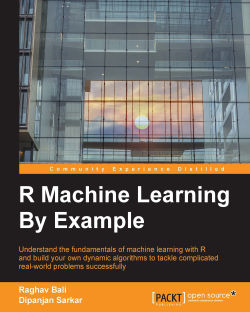# R Machine Learning By Example4.7 (15 reviews total)
By Raghav Bali , Dipanjan Sarkar

• Constantly updated with 100+ new titles each month
• Breadth and depth in over 1,000+ technologies

Data science and machine learning are some of the top buzzwords in the technical world today. From retail stores to Fortune 500 companies, everyone is working hard to making machine learning give them data-driven insights to grow their business. With powerful data manipulation features, machine learning packages, and an active developer community, R empowers users to build sophisticated machine learning systems to solve real-world data problems.

This book takes you on a data-driven journey that starts with the very basics of R and machine learning and gradually builds upon the concepts to work on projects that tackle real-world problems.

You’ll begin by getting an understanding of the core concepts and definitions required to appreciate machine learning algorithms and concepts. Building upon the basics, you will then work on three different projects to apply the concepts of machine learning, following current trends and cover major algorithms as well as popular R packages in detail. These projects have been neatly divided into six different chapters covering the worlds of e-commerce, finance, and social-media, which are at the very core of this data-driven revolution. Each of the projects will help you to understand, explore, visualize, and derive insights depending upon the domain and algorithms.

Through this book, you will learn to apply the concepts of machine learning to deal with data-related problems and solve them using the powerful yet simple language, R.

Publication date:
March 2016
Publisher
Packt
Pages
340
ISBN
9781784390846

## Chapter 1. Getting Started with R and Machine Learning

This introductory chapter will get you started with the basics of R which include various constructs, useful data structures, loops and vectorization. If you are already an R wizard, you can skim through these sections and dive right into the next part which talks about what machine learning actually represents as a domain and the main areas it encompasses. We will also talk about different machine learning techniques and algorithms used in each area. Finally, we will conclude by looking at some of the most popular machine learning packages in R, some of which we will be using in the subsequent chapters.

If you are a data or machine learning enthusiast, surely you would have heard by now that being a data scientist is referred to as the sexiest job of the 21st century by Harvard Business Review.

### Note

There is a huge demand in the current market for data scientists, primarily because their main job is to gather crucial insights and information from both unstructured and structured data to help their business and organization grow strategically.

Some of you might be wondering how machine learning or R relate to all this! Well, to be a successful data scientist, one of the major tools you need in your toolbox is a powerful language capable of performing complex statistical calculations and working with various types of data and building models which help you get previously unknown insights and R is the perfect language for that! Machine learning forms the foundation of the skills you need to build to become a data analyst or data scientist, this includes using various techniques to build models to get insights from data.

This book will provide you with some of the essential tools you need to be well versed with both R and machine learning by not only looking at concepts but also applying those concepts in real-world examples. Enough talk; now let's get started on our journey into the world of machine learning with R!

In this chapter, we will cover the following aspects:

• Delving into the basics of R

• Understanding the data structures in R

• Working with functions

• Controlling code flow

• Taking further steps with R

• Understanding machine learning basics

• Familiarizing yourself with popular machine learning packages in R

## Delving into the basics of R

It is assumed here that you are at least familiar with the basics of R or have worked with R before. Hence, we won't be talking much about downloading and installations. There are plenty of resources on the web which provide a lot of information on this. I recommend that you use RStudio which is an Integrated Development Environment (IDE), which is much better than the base R Graphical User Interface (GUI). You can visit https://www.rstudio.com/ to get more information about it.

### Note

For details about the R project, you can visit https://www.r-project.org/ to get an overview of the language. Besides this, R has a vast arsenal of wonderful packages at its disposal and you can view everything related to R and its packages at https://cran.r-project.org/ which contains all the archives.

You must already be familiar with the R interactive interpreter, often called a Read-Evaluate-Print Loop (REPL). This interpreter acts like any command line interface which asks for input and starts with a `>` character, which indicates that R is waiting for your input. If your input spans multiple lines, like when you are writing a function, you will see a `+` prompt in each subsequent line, which means that you didn't finish typing the complete expression and R is asking you to provide the rest of the expression.

It is also possible for R to read and execute complete files containing commands and functions which are saved in files with an `.R` extension. Usually, any big application consists of several `.R` files. Each file has its own role in the application and is often called as a module. We will be exploring some of the main features and capabilities of R in the following sections.

### Using R as a scientific calculator

The most basic constructs in R include variables and arithmetic operators which can be used to perform simple mathematical operations like a calculator or even complex statistical calculations.

```> 5 + 6
 11
> 3 * 2
 6
> 1 / 0
 Inf
```

Remember that everything in R is a vector. Even the output results indicated in the previous code snippet. They have a leading  symbol indicating it is a vector of size `1`.

You can also assign values to variables and operate on them just like any other programming language.

```> num <- 6
> num ^ 2
 36
> num
 6     # a variable changes value only on re-assignment
> num <- num ^ 2 * 5 + 10 / 3
> num
 183.3333
```

### Operating on vectors

The most basic data structure in R is a vector. Basically, anything in R is a vector, even if it is a single number just like we saw in the earlier example! A vector is basically a sequence or a set of values. We can create vectors using the `:` operator or the `c` function which concatenates the values to create a vector.

```> x <- 1:5
> x
 1 2 3 4 5
> y <- c(6, 7, 8 ,9, 10)
> y
  6  7  8  9 10
> z <- x + y
> z
  7  9 11 13 15
```

You can clearly in the previous code snippet, that we just added two vectors together without using any loop, using just the `+` operator. This is known as vectorization and we will be discussing more about this later on. Some more operations on vectors are shown next:

```> c(1,3,5,7,9) * 2
  2  6 10 14 18
> c(1,3,5,7,9) * c(2, 4)
  2 12 10 28 18 # here the second vector gets recycled
```

Output: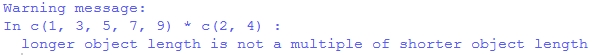```> factorial(1:5)
   1   2   6  24 120
> exp(2:10)   # exponential function
     7.389056    20.085537    54.598150   148.413159   403.428793  1096.633158
  2980.957987  8103.083928 22026.465795
> cos(c(0, pi/4))   # cosine function
 1.0000000 0.7071068
> sqrt(c(1, 4, 9, 16))
 1 2 3 4
> sum(1:10)
 55
```

You might be confused with the second operation where we tried to multiply a smaller vector with a bigger vector but we still got a result! If you look closely, R threw a warning also. What happened in this case is, since the two vectors were not equal in size, the smaller vector in this case `c(2, 4)` got recycled or repeated to become `c(2, 4, 2, 4, 2)` and then it got multiplied with the first vector `c(1, 3, 5, 7 ,9)` to give the final result vector, `c(2, 12, 10, 28, 18)`. The other functions mentioned here are standard functions available in base R along with several other functions.

### Tip

You can download the example code files for this book from your account at http://www.packtpub.com. If you purchased this book elsewhere, you can visit http://www.packtpub.com/support and register to have the files e-mailed directly to you.

• Hover the mouse pointer on the SUPPORT tab at the top

• Enter the name of the book in the Search box

• Select the book for which you're looking to download the code files

• Choose from the drop-down menu where you purchased this book from

• WinRAR / 7-Zip for Windows

• Zipeg / iZip / UnRarX for Mac

• 7-Zip / PeaZip for Linux

### Special values

Since you will be dealing with a lot of messy and dirty data in data analysis and machine learning, it is important to remember some of the special values in R so that you don't get too surprised later on if one of them pops up.

```> 1 / 0
 Inf
> 0 / 0
 NaN
> Inf / NaN
 NaN
> Inf / Inf
 NaN
> log(Inf)
 Inf
> Inf + NA
 NA
```

The main values which should concern you here are `Inf` which stands for Infinity, `NaN` which is Not a Number, and `NA` which indicates a value that is missing or Not Available. The following code snippet shows some logical tests on these special values and their results. Do remember that `TRUE` and `FALSE` are logical data type values, similar to other programming languages.

```> vec <- c(0, Inf, NaN, NA)
> is.finite(vec)
  TRUE FALSE FALSE FALSE
> is.nan(vec)
 FALSE FALSE  TRUE FALSE
> is.na(vec)
 FALSE FALSE  TRUE  TRUE
> is.infinite(vec)
 FALSE  TRUE FALSE FALSE
```

The functions are pretty self-explanatory from their names. They clearly indicate which values are finite, which are finite and checks for `NaN` and `NA` values respectively. Some of these functions are very useful when cleaning dirty data.

## Data structures in R

Here we will be looking at the most useful data structures which exist in R and use using them on some fictional examples to get a better grasp on their syntax and constructs. The main data structures which we will be covering here include:

• Vectors

• Arrays and matrices

• Lists

• Data frames

These data structures are used widely inside R as well as by various R packages and functions, including machine learning functions and algorithms which we will be using in the subsequent chapters. So it is essential to know how to use these data structures to work with data efficiently.

### Vectors

Just like we mentioned briefly in the previous sections, vectors are the most basic data type inside R. We use vectors to represent anything, be it input or output. We previously saw how we create vectors and apply mathematical operations on them. We will see some more examples here.

#### Creating vectors

Here we will look at ways to initialize vectors, some of which we had also worked on previously, using operators such as `:` and functions such as `c`. In the following code snippet, we will use the `seq` family of functions to initialize vectors in different ways.

```> c(2.5:4.5, 6, 7, c(8, 9, 10), c(12:15))
  2.5  3.5  4.5  6.0  7.0  8.0  9.0 10.0 12.0 13.0 14.0 15.0
> vector("numeric", 5)
 0 0 0 0 0
> vector("logical", 5)
 FALSE FALSE FALSE FALSE FALSE
> logical(5)
 FALSE FALSE FALSE FALSE FALSE
> # seq is a function which creates sequences
> seq.int(1,10)
  1  2  3  4  5  6  7  8  9 10
> seq.int(1,10,2)
 1 3 5 7 9
> seq_len(10)
  1  2  3  4  5  6  7  8  9 10
```

#### Indexing and naming vectors

One of the most important operations we can do on vectors involves subsetting and indexing vectors to access specific elements which are often useful when we want to run some code only on specific data points. The following examples show some ways in which we can index and subset vectors:

```> vec <- c("R", "Python", "Julia", "Haskell", "Java", "Scala")
> vec
 "R"
> vec[2:4]
> vec[c(1, 3, 5)]
 "R"     "Julia" "Java"
> nums <- c(5, 8, 10, NA, 3, 11)
> nums
  5  8 10 NA  3 11
> which.min(nums)   # index of the minimum element
 5
> which.max(nums)   # index of the maximum element
 6
> nums[which.min(nums)]  # the actual minimum element
 3
> nums[which.max(nums)]  # the actual maximum element
 11
```

Now we look at how we can name vectors. This is basically a nifty feature in R where you can label each element in a vector to make it more readable or easy to interpret. There are two ways this can be done, which are shown in the following examples:

```> c(first=1, second=2, third=3, fourth=4, fifth=5)
```

Output: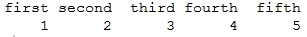```> positions <- c(1, 2, 3, 4, 5)
> names(positions)
NULL
> names(positions) <- c("first", "second", "third", "fourth", "fifth")
> positions
```

Output:```> names(positions)
 "first"  "second" "third"  "fourth" "fifth"
> positions[c("second", "fourth")]
```

Output: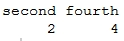Thus, you can see, it becomes really useful to annotate and name vectors sometimes, and we can also subset and slice vectors using element names rather than values.

### Arrays and matrices

Vectors are one dimensional data structures, which means that they just have one dimension and we can get the number of elements they have using the `length` property. Do remember that arrays may also have a similar meaning in other programming languages, but in R they have a slightly different meaning. Basically, arrays in R are data structures which hold the data having multiple dimensions. Matrices are just a special case of generic arrays having two dimensions, namely represented by properties `rows` and `columns`. Let us look at some examples in the following code snippets in the accompanying subsection.

#### Creating arrays and matrices

First we will create an array that has three dimensions. Now it is easy to represent two dimensions in your screen, but to go one dimension higher, there are special ways in which R transforms the data. The following example shows how R fills the data (column first) in each dimension and shows the final output for a 4x3x3 array:

```> three.dim.array <- array(
+     1:32,    # input data
+     dim = c(4, 3, 3),   # dimensions
+     dimnames = list(    # names of dimensions
+         c("row1", "row2", "row3", "row4"),
+         c("col1", "col2", "col3"),
+         c("first.set", "second.set", "third.set")
+     )
+ )
> three.dim.array
```

Output: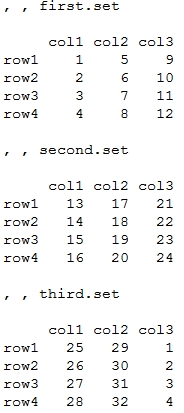Like I mentioned earlier, a matrix is just a special case of an array. We can create a matrix using the `matrix` function, shown in detail in the following example. Do note that we use the parameter `byrow` to fill the data row-wise in the matrix instead of R's default column-wise fill in any array or matrix. The `ncol` and `nrow` parameters stand for number of columns and rows respectively.

```> mat <- matrix(
+     1:24,   # data
+     nrow = 6,  # num of rows
+     ncol = 4,  # num of columns
+     byrow = TRUE,  # fill the elements row-wise
+ )
> mat
```

Output: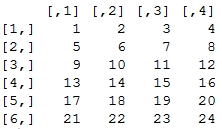#### Names and dimensions

Just like we named vectors and accessed element names, will perform similar operations in the following code snippets. You have already seen the use of the `dimnames` parameter in the preceding examples. Let us look at some more examples as follows:

```> dimnames(three.dim.array)
```

Output: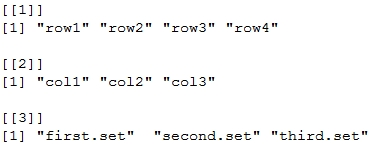```> rownames(three.dim.array)
 "row1" "row2" "row3" "row4"
> colnames(three.dim.array)
 "col1" "col2" "col3"
> dimnames(mat)
NULL
> rownames(mat)
NULL
> rownames(mat) <- c("r1", "r2", "r3", "r4", "r5", "r6")
> colnames(mat) <- c("c1", "c2", "c3", "c4")
> dimnames(mat)
```

Output: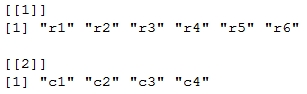```> mat
```

Output: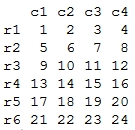To access details of dimensions related to arrays and matrices, there are special functions. The following examples show the same:

```> dim(three.dim.array)
 4 3 3
> nrow(three.dim.array)
 4
> ncol(three.dim.array)
 3
> length(three.dim.array)  # product of dimensions
 36
> dim(mat)
 6 4
> nrow(mat)
 6
> ncol(mat)
 4
> length(mat)
 24
```

#### Matrix operations

A lot of machine learning and optimization algorithms deal with matrices as their input data. In the following section, we will look at some examples of the most common operations on matrices.

We start by initializing two matrices and then look at ways of combining the two matrices using functions such as `c` which returns a vector, `rbind` which combines the matrices by `rows,` and `cbind` which does the same by columns.

```> mat1 <- matrix(
+     1:15,
+     nrow = 5,
+     ncol = 3,
+     byrow = TRUE,
+     dimnames = list(
+         c("M1.r1", "M1.r2", "M1.r3", "M1.r4", "M1.r5")
+         ,c("M1.c1", "M1.c2", "M1.c3")
+     )
+ )
> mat1
```

Output: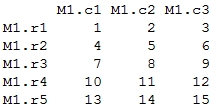```> mat2 <- matrix(
+     16:30,
+     nrow = 5,
+     ncol = 3,
+     byrow = TRUE,
+     dimnames = list(
+         c("M2.r1", "M2.r2", "M2.r3", "M2.r4", "M2.r5"),
+         c("M2.c1", "M2.c2", "M2.c3")
+     )
+ )
> mat2
```

Output: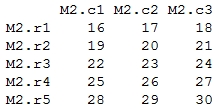```> rbind(mat1, mat2)
```

Output: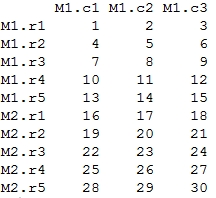```> cbind(mat1, mat2)
```

Output: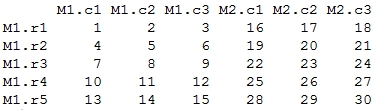```> c(mat1, mat2)
```

Output: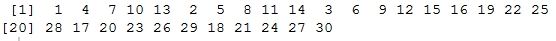Now we look at some of the important arithmetic operations which can be performed on matrices. Most of them are quite self-explanatory from the following syntax:

```> mat1 + mat2   # matrix addition
```

Output: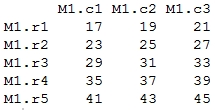```> mat1 * mat2  # element-wise multiplication
```

Output: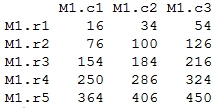```> tmat2 <- t(mat2)  # transpose
> tmat2
```

Output: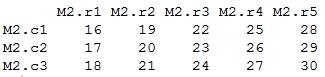```> mat1 %*% tmat2   # matrix inner product
```

Output: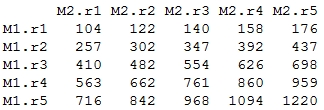```> m <- matrix(c(5, -3, 2, 4, 12, -1, 9, 14, 7), nrow = 3, ncol = 3)
> m
```

Output: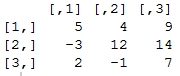```> inv.m <- solve(m)  # matrix inverse
> inv.m
```

Output: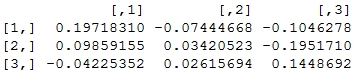```> round(m %*% inv.m) # matrix * matrix_inverse = identity matrix
```

Output: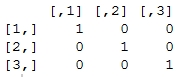The preceding arithmetic operations are just some of the most popular ones amongst the vast number of functions and operators which can be applied to matrices. This becomes useful, especially in areas such as linear optimization.

### Lists

Lists are a special case of vectors where each element in the vector can be of a different type of data structure or even simple data types. It is similar to the lists in the Python programming language in some aspects, if you have used it before, where the lists indicate elements which can be of different types and each have a specific index in the list. In R, each element of a list can be as simple as a single element or as complex as a whole matrix, a function, or even a vector of strings.

#### Creating and indexing lists

We will get started with looking at some common methods to create and initialize lists in the following examples. Besides that, we will also look at how we can access some of these list elements for further computations. Do remember that each element in a list can be a simple primitive data type or even complex data structures or functions.

```> list.sample <- list(
+     1:5,
+     c("first", "second", "third"),
+     c(TRUE, FALSE, TRUE, TRUE),
+     cos,
+     matrix(1:9, nrow = 3, ncol = 3)
+ )
> list.sample
```

Output: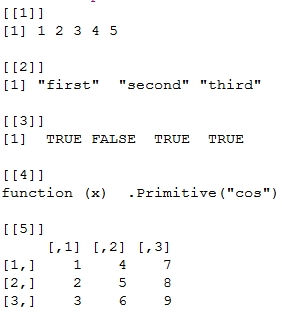```> list.with.names <- list(
+     even.nums = seq.int(2,10,2),
+     odd.nums  = seq.int(1,10,2),
+     languages = c("R", "Python", "Julia", "Java"),
+     cosine.func = cos
+ )
> list.with.names
```

Output: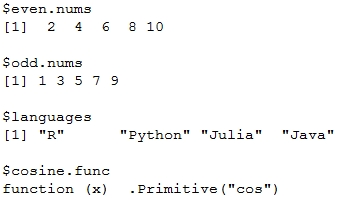```> list.with.names\$cosine.func
function (x)  .Primitive("cos")
> list.with.names\$cosine.func(pi)
 -1
>
> list.sample[]
function (x)  .Primitive("cos")
> list.sample[](pi)
 -1
>
> list.with.names\$odd.nums
 1 3 5 7 9
> list.sample[]
 1 2 3 4 5
> list.sample[]
  TRUE FALSE  TRUE  TRUE
```

You can see from the preceding examples how easy it is to access any element of the list and use it for further computations, such as the `cos` function.

#### Combining and converting lists

Now we will take a look at how to combine several lists together into one single list in the following examples:

```> l1 <- list(
+     nums = 1:5,
+     chars = c("a", "b", "c", "d", "e"),
+     cosine = cos
+ )
> l2 <- list(
+     languages = c("R", "Python", "Java"),
+     months = c("Jan", "Feb", "Mar", "Apr"),
+     sine = sin
+ )
> # combining the lists now
> l3 <- c(l1, l2)
> l3
```

Output: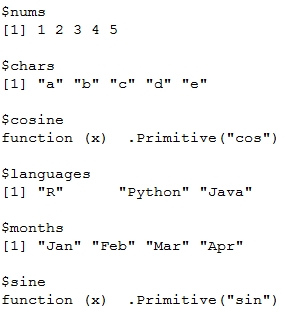It is very easy to convert lists in to vectors and vice versa. The following examples show some common ways we can achieve this:

```> l1 <- 1:5
> class(l1)
 "integer"
> list.l1 <- as.list(l1)
> class(list.l1)
 "list"
> list.l1
```

Output: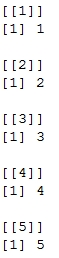```> unlist(list.l1)
 1 2 3 4 5
```

### Data frames

Data frames are special data structures which are typically used for storing data tables or data in the form of spreadsheets, where each column indicates a specific attribute or field and the rows consist of specific values for those columns. This data structure is extremely useful in working with datasets which usually have a lot of fields and attributes.

#### Creating data frames

We can create data frames easily using the `data.frame` function. We will look at some following examples to illustrate the same with some popular superheroes:

```> df <- data.frame(
+     real.name = c("Bruce Wayne", "Clark Kent", "Slade Wilson", "Tony Stark", "Steve Rogers"),
+     superhero.name = c("Batman", "Superman", "Deathstroke", "Iron Man", "Capt. America"),
+     franchise = c("DC", "DC", "DC", "Marvel", "Marvel"),
+     team = c("JLA", "JLA", "Suicide Squad", "Avengers", "Avengers"),
+     origin.year = c(1939, 1938, 1980, 1963, 1941)
+ )
> df
```

Output: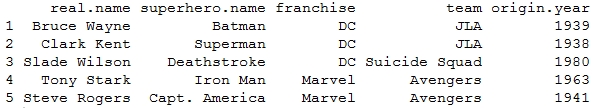```> class(df)
 "data.frame"
> str(df)
```

Output: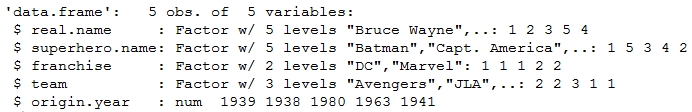```> rownames(df)
 "1" "2" "3" "4" "5"
> colnames(df)
```

Output: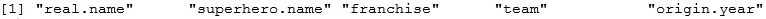```> dim(df)
 5 5
```

The `str` function talks in detail about the structure of the data frame where we see details about the data present in each column of the data frame. There are a lot of datasets readily available in R base which you can directly load and start using. One of them is shown next. The `mtcars` dataset has information about various automobiles, which was extracted from the Motor Trend U.S. Magazine of 1974.

```> head(mtcars)   # one of the datasets readily available in R
```

Output: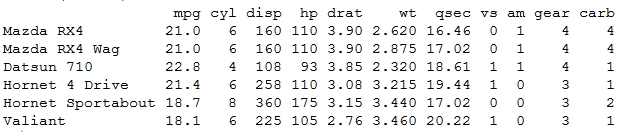#### Operating on data frames

There are a lot of operations we can do on data frames, such as merging, combining, slicing, and transposing data frames. We will look at some of the important data frame operations in the following examples.

It is really easy to index and subset specific data inside data frames using simplex indexes and functions such as `subset`.

```> df[2:4,]
```

Output: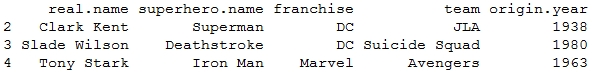```> df[2:4, 1:2]
```

Output: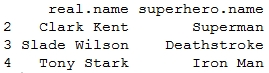```> subset(df, team=="JLA", c(real.name, superhero.name, franchise))
```

Output: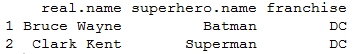```> subset(df, team %in% c("Avengers","Suicide Squad"), c(real.name, superhero.name, franchise))
```

Output: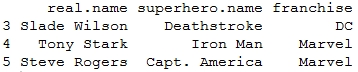We will now look at some more complex operations, such as combining and merging data frames.

```> df1 <- data.frame(
+     id = c('emp001', 'emp003', 'emp007'),
+     name = c('Harvey Dent', 'Dick Grayson', 'James Bond'),
+     alias = c('TwoFace', 'Nightwing', 'Agent 007')
+ )
>
> df2 <- data.frame(
+     id = c('emp001', 'emp003', 'emp007'),
+     location = c('Gotham City', 'Gotham City', 'London'),
+     speciality = c('Split Persona', 'Expert Acrobat', 'Gadget Master')
+ )
> df1
```

Output: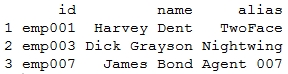```> df2
```

Output: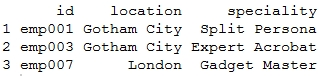```> rbind(df1, df2)   # not possible since column names don't match
Error in match.names(clabs, names(xi)) :
names do not match previous names
> cbind(df1, df2)
```

Output: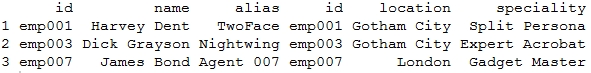```> merge(df1, df2, by="id")
```

Output: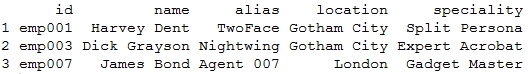From the preceding operations it is evident that `rbind` and `cbind` work in the same way as we saw previously with arrays and matrices. However, merge lets you merge the data frames in the same way as you join various tables in relational databases.

## Working with functions

Next up, we will be looking at functions, which is a technique or methodology to easily structure and modularize your code, specifically lines of code which perform specific tasks, so that you can execute them whenever you need them without writing them again and again. In R, functions are basically treated as just another data type and you can assign functions, manipulate them as and when needed, and also pass them as arguments to other functions. We will be exploring all this in the following section.

### Built-in functions

R consists of several functions which are available in the R-base package and, as you install more packages, you get more functionality, which is made available in the form of functions. We will look at a few built-in functions in the following examples:

```> sqrt(5)
 2.236068
> sqrt(c(1,2,3,4,5,6,7,8,9,10))
 1.000000 1.414214 1.732051 2.000000 2.236068 2.449490 2.645751      2.828427 3.000000 3.162278
> # aggregating functions
> mean(c(1,2,3,4,5,6,7,8,9,10))
 5.5
> median(c(1,2,3,4,5,6,7,8,9,10))
 5.5
```

You can see from the preceding examples that functions such as `mean`, `median,` and `sqrt` are built-in and can be used anytime when you start R, without loading any other packages or defining the functions explicitly.

### User-defined functions

The real power lies in the ability to define your own functions based on different operations and computations you want to perform on the data and making R execute those functions just in the way you intend them to work. Some illustrations are shown as follows:

```square <- function(data){
return (data^2)
}
> square(5)
 25
> square(c(1,2,3,4,5))
  1  4  9 16 25
point <- function(xval, yval){
return (c(x=xval,y=yval))
}
> p1 <- point(5,6)
> p2 <- point(2,3)
>
> p1
x y
5 6
> p2
x y
2 3
```

As we saw in the previous code snippet, we can define functions such as `square` which computes the square of a single number or even a vector of numbers using the same code. Functions such as point are useful to represent specific entities which represent `points` in the two-dimensional co-ordinate space. Now we will be looking at how to use the preceding functions together.

### Passing functions as arguments

When you define any function, you can also pass other functions to it as arguments if you intend to use them inside your function to perform some complex computations. This reduces the complexity and redundancy of the code. The following example computes the Euclidean distance between two points using the `square` function defined earlier, which is passed as an argument:

```> # defining the function
euclidean.distance <- function(point1, point2, square.func){
distance <- sqrt(
as.integer(
square.func(point1['x'] - point2['x'])
) +
as.integer(
square.func(point1['y'] - point2['y'])
)
)
return (c(distance=distance))
}
> # executing the function, passing square as argument
> euclidean.distance(point1 = p1, point2 = p2, square.func = square)
distance
4.242641
> euclidean.distance(point1 = p2, point2 = p1, square.func = square)
distance
4.242641
> euclidean.distance(point1 = point(10, 3), point2 = point(-4, 8), square.func = square)
distance
14.86607
```

Thus, you can see that with functions you can define a specific function once and execute it as many times as you need.

## Controlling code flow

This section covers areas related to controlling the execution of your code. Using specific constructs such as `if-else` and `switch`, you can execute code conditionally. Constructs like `for`, `while`, and `repeat`, and `help` in executing the same code multiple times which is also known as looping. We will be exploring all these constructs in the following section.

### Working with if, if-else, and ifelse

There are several constructs which help us in executing code conditionally. This is especially useful when we don't want to execute a bunch of statements one after the other sequentially but execute the code only when it meets or does not meet specific conditions. The following examples illustrate the same:

```> num = 5
> if (num == 5){
+     cat('The number was 5')
+ }
The number was 5
>
> num = 7
>
> if (num == 5){
+     cat('The number was 5')
+ } else{
+     cat('The number was not 5')
+ }
The number was not 5
>
> if (num == 5){
+     cat('The number was 5')
+ } else if (num == 7){
+     cat('The number was 7')
+ } else{
+     cat('No match found')
+ }
The number was 7
> ifelse(num == 5, "Number was 5", "Number was not 5")
 "Number was not 5"
```

### Working with switch

The `switch` function is especially useful when you have to match an expression or argument to several conditions and execute only if there is a specific match. This becomes extremely messy when implemented with the `if-else` constructs but is much more elegant with the `switch` function, as we will see next:

```> switch(
+ "first",
+ first = "1st",
+ second = "2nd",
+ third = "3rd",
+ "No position"
+ )
 "1st"
>
> switch(
+ "third",
+ first = "1st",
+ second = "2nd",
+ third = "3rd",
+ "No position"
+ )
 "3rd"
> # when no match, default statement executes
> switch(
+ "fifth",
+ first = "1st",
+ second = "2nd",
+ third = "3rd",
+ "No position"
+ )
 "No position"
```

### Loops

Loops are an excellent way to execute code segments repeatedly when needed. Vectorization constructs are, however, more optimized than loops for working on larger data sets, but we will see that later in this chapter. For now, you should remember that there are three types of loops in R, namely, `for`, `while`, and `repeat`. We will look at all of them in the following examples:

```> # for loop
> for (i in 1:10){
+     cat(paste(i," "))
+ }
1  2  3  4  5  6  7  8  9  10
>
> sum = 0
> for (i in 1:10){
+     sum <- sum + i
+ }
> sum
 55
>
> # while loop
> count <- 1
> while (count <= 10){
+     cat(paste(count, " "))
+     count <- count + 1
+ }
1  2  3  4  5  6  7  8  9  10
>
> # repeat infinite loop
> count = 1
> repeat{
+     cat(paste(count, " "))
+     if (count >= 10){
+         break  # break off from the infinite loop
+     }
+     count <- count + 1
+ }
1  2  3  4  5  6  7  8  9  10
```

We heard the term vectorized earlier when we talked about operating on vectors without using loops. While looping is a great way to iterate through vectors and perform computations, it is not very efficient when we deal with what is known as Big Data. In this case, R provides some advanced constructs which we will be looking at in this section. We will be covering the following functions:

• `lapply`: Loops over a list and evaluates a function on each element

• `sapply`: A simplified version of lapply

• `apply`: Evaluates a function on the boundaries or margins of an array

• `tapply`: Evaluates a function over subsets of a vector

• `mapply`: A multivariate version of lapply

### lapply and sapply

Like we mentioned earlier, `lapply` takes a list and a function as input and evaluates that function over each element of the list. If the input list is not a list, it is converted into a list using the `as.list` function before the output is returned. It is much faster than a normal loop because the actual looping is done internally using C code. We look at its implementation and an example in the following code snippet:

```> # lapply function definition
> lapply
function (X, FUN, ...)
{
FUN <- match.fun(FUN)
if (!is.vector(X) || is.object(X))
X <- as.list(X)
.Internal(lapply(X, FUN))
}
<bytecode: 0x00000000003e4f68>
<environment: namespace:base>
> # example
> nums <- list(l1=c(1,2,3,4,5,6,7,8,9,10), l2=1000:1020)
> lapply(nums, mean)
```

Output: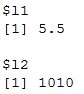Coming to `sapply`, it is similar to `lapply` except that it tries to simplify the results wherever possible. For example, if the final result is such that every element is of length `1`, it returns a vector, if the length of every element in the result is the same but more than 1, a matrix is returned, and if it is not able to simplify the results, we get the same result as `lapply`. We illustrate the same with the following example:

```> data <- list(l1=1:10, l2=runif(10), l3=rnorm(10,2))
> data
```

Output: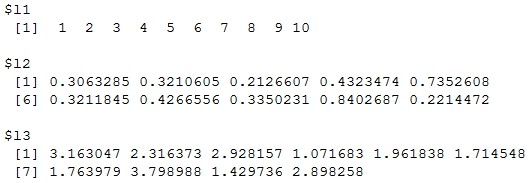```>
> lapply(data, mean)
```

Output: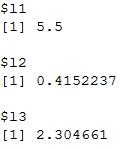```> sapply(data, mean)
```

Output: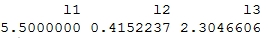### apply

The `apply` function is used to evaluate a function over the margins or boundaries of an array; for instance, applying aggregate functions on the rows or columns of an array. The `rowSums`, `rowMeans`, `colSums,` and `colMeans` functions also use `apply` internally but are much more optimized and useful when operating on large arrays. We will see all the preceding constructs in the following example:

```> mat <- matrix(rnorm(20), nrow=5, ncol=4)
> mat
```

Output: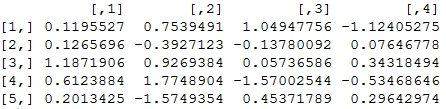```> # row sums
> apply(mat, 1, sum)
  0.79786959  0.53900665 -2.36486927 -1.28221227  0.06701519
> rowSums(mat)
  0.79786959  0.53900665 -2.36486927 -1.28221227  0.06701519
> # row means
> apply(mat, 1, mean)
  0.1994674  0.1347517 -0.5912173 -0.3205531  0.0167538
> rowMeans(mat)
  0.1994674  0.1347517 -0.5912173 -0.3205531  0.0167538
>
> # col sums
> apply(mat, 2, sum)
 -0.6341087  0.3321890 -2.1345245  0.1932540
> colSums(mat)
 -0.6341087  0.3321890 -2.1345245  0.1932540
> apply(mat, 2, mean)
 -0.12682173  0.06643781 -0.42690489  0.03865079
> colMeans(mat)
 -0.12682173  0.06643781 -0.42690489  0.03865079
>
> # row quantiles
> apply(mat, 1, quantile, probs=c(0.25, 0.5, 0.75))
```

Output:Thus you can see how easy it is to apply various statistical functions on matrices without using loops at all.

### tapply

The function `tapply` is used to evaluate a function over the subsets of any vector. This is similar to applying the `GROUP BY` construct in SQL if you are familiar with using relational databases. We illustrate the same in the following examples:

```> data <- c(1:10, rnorm(10,2), runif(10))
> data
```

Output: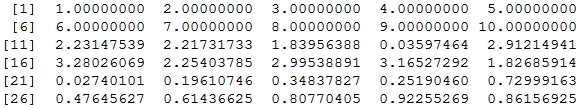```> groups <- gl(3,10)
> groups
 1 1 1 1 1 1 1 1 1 1 2 2 2 2 2 2 2 2 2 2 3 3 3 3 3 3 3 3 3 3
Levels: 1 2 3
> tapply(data, groups, mean)
```

Output: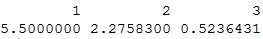```> tapply(data, groups, mean, simplify = FALSE)
```

Output: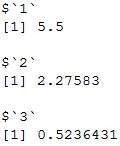```> tapply(data, groups, range)
```

Output: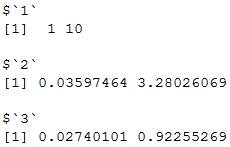### mapply

The `mapply` function is a multivariate version of `lapply` and is used to evaluate a function in parallel over sets of arguments. A simple example is if we have to build a list of vectors using the `rep` function, we have to write it multiple times. However, with `mapply` we can achieve the same in a more elegant way as illustrated next:

```> list(rep(1,4), rep(2,3), rep(3,2), rep(4,1))
```

Output: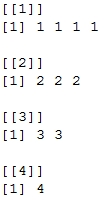```> mapply(rep, 1:4, 4:1)
```

Output:## Next steps with R

Before we dive into machine learning, it will be useful to pause for a moment, take a deep breath, and contemplate on what you have learnt so far. This quick yet detailed refresher of R will help you a lot in the upcoming chapters. However, there are two more things which we must go through quickly. They are how to get help in R and how to work with various packages in R.

### Getting help

By now, you must have figured out that there are thousands of functions and constructs in R and it is impossible to remember what each of them actually does and you don't have to either! R provides many intuitive ways to get help regarding any function, package, or data structure. To start with, you can run the `help.start()` function at the `R` command prompt, which will start a manual browser. Here you will get detailed information regarding R which includes manuals, references, and other material. The following command shows the contents of `help.start()` as shown in the screenshot following the command, which you can use to navigate further and get more help:

```> help.start()
```

If nothing happens, you should open `http://127.0.0.1:31850/doc/html/index.html` yourself.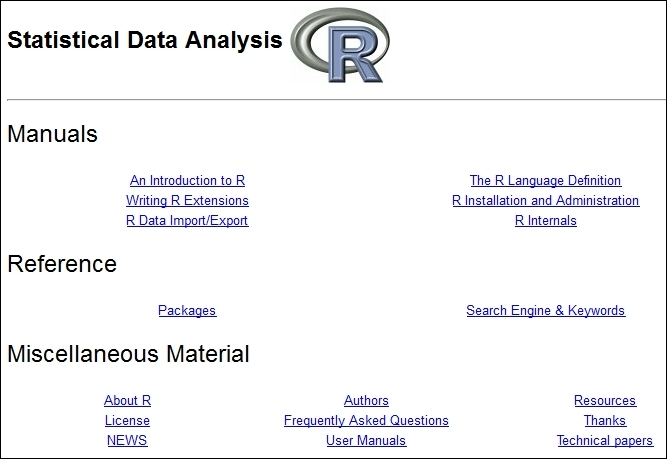To get help on any particular function or construct in R, if you know the function's name, you can get help using the help function or the `?` operator in combination with the function name. For example, if you want help regarding the `apply` function, just type `help("apply")` or `?apply` to get detailed information regarding the `apply` function. This easy mechanism for getting help in R increases your productivity and makes working with R a pleasant experience. Often, you won't quite remember the exact name of the function you intend to use but you might have a vague idea of what its name might be. R has a help feature for this purpose too, where you can use the `help.search` function or the `??` operator, in combination with the function name. For example, you can use `??apply` to get more information on the apply function.

### Handling packages

There are thousands and thousands of packages containing a wide variety of capabilities available on CRAN (Comprehensive R Archive Network), which is a repository hosting all these packages. To download any package from CRAN, all you have to do is run the `install.packages` function passing the package name as a parameter, like `install.packages("caret")`. Once the package is downloaded and installed, you can load it into your current R session using the `library` function. To load the package `caret`, just type `library(caret)` and it should be readily available for use. The `require` function has similar functionality to load a specific package and is used specially inside functions in a similar way by typing `require(caret)` to load the `caret` package. The only difference between `require` and `library` is that, in case the specific package is not found, `library` will show an error but `require` will continue the execution of code without showing any error. However, if there is a dependency call to that package then your code will definitely throw an error.

## Machine learning basics

Now that you have refreshed your memory about R, we will be talking about the basics of what machine learning is, how it is used today, and what are the main areas inside machine learning. This section intends to provide an overview into machine learning which will help in paving the way to the next chapter where we will be exploring it in more depth.

### Machine learning – what does it really mean?

Machine learning does not have just one distinct textbook definition because it is a field which encompasses and borrows concepts and techniques from several other areas in computer science. It is also taught as an academic course in universities and has recently gained more prominence, with machine learning and data science being widely adopted online, in the form of educational videos, courses, and training. Machine learning is basically an intersection of elements from the fields of computer science, statistics, and mathematics, which uses concepts from artificial intelligence, pattern detection, optimization, and learning theory to develop algorithms and techniques which can learn from and make predictions on data without being explicitly programmed.

The learning here refers to the ability to make computers or machines intelligent based on the data and algorithms which we provide to them so that they start detecting patterns and insights from the provided data. This learning ensures that machines can detect patterns on data fed to it without explicitly programming them every time. The initial data or observations are fed to the machine and the machine learning algorithm works on that data to generate some output which can be a prediction, a hypothesis, or even some numerical result. Based on this output, there can be feedback mechanisms to our machine learning algorithm to improve our results. This whole system forms a machine learning model which can be used directly on completely new data or observations to get results from it without needing to write any separate algorithm again to work on that data.

### Machine learning – how is it used in the world?

You might be wondering how on earth some algorithms or code can be used in the real world. It turns out they are used in a wide variety of use-cases in different verticals. Some examples are as follows:

• Retail: Machine learning is widely used in the retail and e-commerce vertical where each store wants to outperform its competitors.

• Pricing analytics: Machine learning algorithms are used to compare prices for items across various stores so that a store can sell the item at the most competitive price.

• Market basket analysis: They are used for analysis of customer shopping trends and recommendation of products to buy, which we will be covering in Chapter 3, Predicting Customer Shopping Trends with Market Basket Analysis.

• Recommendation engines: They are used to analyze customer purchases, ratings, and satisfaction to recommend products to various users. We will be building some recommendation systems of our own in Chapter 4, Building a Product Recommendation System.

• Web analytics: Analyzes website traffic

• Churn analytics: Predicts customer churn rate

• Healthcare: Machine learning algorithms are used widely in the healthcare vertical for more effective treatment of patients.

• Disease detection and prediction: Used to detect and predict chances of a disease based on the patient's medical history.

• Studying complex structures such as the human brain and DNA to understand the human body's functionality better for more effective treatment.

• Detection and filtering of spam e-mails and messages.

• Predicting election results.

• Fraud detection and prediction. We will be taking a stab at one of the most critical fraud detection problems in Chapters 5, Credit Risk Detection and Prediction – Descriptive Analytics and Chapter 6, Credit Risk Detection and Prediction – Predictive Analytics.

• Text prediction in a messaging application.

• Self-driving cars, planes, and other vehicles.

• Weather, traffic, and crime activity forecasting and prediction.

• Sentiment and emotion analysis, which we will be covering in Chapter 8, Sentiment Analysis of Twitter Data.

The preceding examples just scratch the surface of what machine learning can really do and by now I am sure that you have got a good flavor of the various areas where machine learning is being used extensively.

### Types of machine learning algorithms

As we talked about earlier, to make machines learn, you need machine learning algorithms. Machine learning algorithms are a special class of algorithms which work on data and gather insights from it. The idea is to build a model using a combination of data and algorithms which can then be used to work on new data and derive actionable insights.

Each machine learning algorithm depends on what type of data it can work on and what type of problem are we trying to solve. You might be tempted to learn a couple of algorithms and then try to apply them to every problem you face. Do remember that there is no universal machine learning algorithm which fits all problems. The main input to machine learning algorithms is data which consists of features, where each feature can be described as an attribute of the data set, such as your height, weight, and so on if we were dealing with data related to human beings. Machine learning algorithms can be divided into two main areas, namely supervised and unsupervised learning algorithms.

#### Supervised machine learning algorithms

The supervised learning algorithms are a subset of the family of machine learning algorithms which are mainly used in predictive modeling. A predictive model is basically a model constructed from a machine learning algorithm and features or attributes from training data such that we can predict a value using the other values obtained from the input data. Supervised learning algorithms try to model relationships and dependencies between the target prediction output and the input features such that we can predict the output values for new data based on those relationships which it learned from the previous data sets. The main types of supervised learning algorithms include:

• Classification algorithms: These algorithms build predictive models from training data which have features and class labels. These predictive models in-turn use the features learnt from training data on new, previously unseen data to predict their class labels. The output classes are discrete. Types of classification algorithms include decision trees, random forests, support vector machines, and many more. We will be using several of these algorithms in Chapter 2, Let's Help Machines Learn, Chapter 6, Credit Risk Detection and Prediction – Predictive Analytics, and Chapter 8, Sentiment Analysis of Twitter Data.

• Regression algorithms: These algorithms are used to predict output values based on some input features obtained from the data. To do this, the algorithm builds a model based on features and output values of the training data and this model is used to predict values for new data. The output values in this case are continuous and not discrete. Types of regression algorithms include linear regression, multivariate regression, regression trees, and lasso regression, among many others. We explore some of these in Chapter 2, Let's Help Machines Learn.

#### Unsupervised machine learning algorithms

The unsupervised learning algorithms are the family of machine learning algorithms which are mainly used in pattern detection and descriptive modeling. A descriptive model is basically a model constructed from an unsupervised machine learning algorithm and features from input data similar to the supervised learning process. However, there are no output categories or labels here based on which the algorithm can try to model relationships. These algorithms try to use techniques on the input data to mine for rules, detect patterns, and summarize and group the data points which help in deriving meaningful insights and describe the data better to the users. There is no specific concept of training or testing data here since we do not have any specific relationship mapping and we are just trying to get useful insights and descriptions from the data we are trying to analyze. The main types of unsupervised learning algorithms include:

• Clustering algorithms: The main objective of these algorithms is to cluster or group input data points into different classes or categories using just the features derived from the input data alone and no other external information. Unlike classification, the output labels are not known beforehand in clustering. There are different approaches to build clustering models, such as by using means, medoids, hierarchies, and many more. Some popular clustering algorithms include k-means, k-medoids, and hierarchical clustering. We will look at some clustering algorithms in Chapter 2, Let's Help Machines Learn, and Chapter 7, Social Media Analysis – Analyzing Twitter Data.

• Association rule learning algorithms: These algorithms are used to mine and extract rules and patterns from data sets. These rules explain relationships between different variables and attributes, and also depict frequent item sets and patterns which occur in the data. These rules in turn help discover useful insights for any business or organization from their huge data repositories. Popular algorithms include Apriori and FP Growth. We will be using some of these in Chapter 2, Let's Help Machines Learn, and Chapter 3, Predicting Customer Shopping Trends with Market Basket Analysis.

#### Popular machine learning packages in R

After getting a brief overview of machine learning basics and types of algorithms, you must be getting inquisitive as to how we apply some of these algorithms to solve real world problems using R. It turns out, there are a whole lot of packages in R which are dedicated to just solving machine learning problems. These packages consist of algorithms which are optimized and ready to be used to solve problems. We will list several popular machine learning packages in R, so that you are aware of what tools you might need later on and also feel more familiar with some of these packages when used in the later chapters. Based on usage and functionality, the following R packages are quite popular in solving machine learning problems:

• `caret`: This package (short for classification and regression training) consists of several machine learning algorithms for building predictive models

• `randomForest`: This package deals with implementations of the random forest algorithm for classification and regression

• `rpart`: This package focuses on recursive partitioning and decision trees

• `glmnet`: The main focus of this package is lasso and elastic-net regularized regression models

• `e1071`: This deals with fourier transforms, clustering, support vector machines, and many more supervised and unsupervised algorithms

• `party`: This deals with recursive partitioning

• `arules`: This package is used for association rule learning algorithms

• `recommenderlab`: This is a library to build recommendation engines

• `nnet`: This package enables predictive modeling using neural networks

• `h2o`: It is one of the most popular packages being used in data science these days and offers fast and scalable algorithms including gradient boosting and deep learning

Besides the preceding libraries, there are a ton of other packages out there related to machine learning in R. What matters is choosing the right algorithm and model based on the data and problem in hand.

## Summary

In this chapter, we talked briefly about the journey we will take into the world of machine learning and R. We discussed the basics of R and built a strong foundation of the core constructs and data structures used in R. Then we dived into the world of machine learning by looking at some concepts and algorithms, and how it is used in the world to solve problems. Finally, we ended with a quick glance at some of the most popular machine learning packages in R to get us all familiarized with some handy tools for our machine learning toolbox!

In the next chapter, we will be looking in depth about machine learning concepts and algorithms which will help us make the machines learn something!

• ##### Raghav Bali

Raghav Bali is a Data Scientist at Optum (United Health Group). His work involves research & development of enterprise level solutions based on Machine Learning, Deep Learning and Natural Language Processing for Healthcare & Insurance related use cases. In his previous role at Intel, he was involved in enabling proactive data driven IT initiatives. He has also worked in ERP and finance domains with some of the leading organizations in the world. Raghav has also authored multiple books with leading publishers. Raghav has a master’s degree (gold medalist) in Information Technology from International Institute of Information Technology, Bangalore. Raghav loves reading and is a shutterbug capturing moments when he isn’t busy solving problems.

Browse publications by this author
• ##### Dipanjan Sarkar

Dipanjan (DJ) Sarkar is a Data Scientist at Intel, leveraging data science, machine learning, and deep learning to build large-scale intelligent systems. He holds a master of technology degree with specializations in Data Science and Software Engineering. He has been an analytics practitioner for several years now, specializing in machine learning, NLP, statistical methods, and deep learning. He is passionate about education and also acts as a Data Science Mentor at various organizations like Springboard, helping people learn data science. He is also a key contributor and editor for Towards Data Science, a leading online journal on AI and Data Science. He has also authored several books on R, Python, machine learning, NLP, and deep learning.

Browse publications by this author

### Latest Reviews

(15 reviews total)